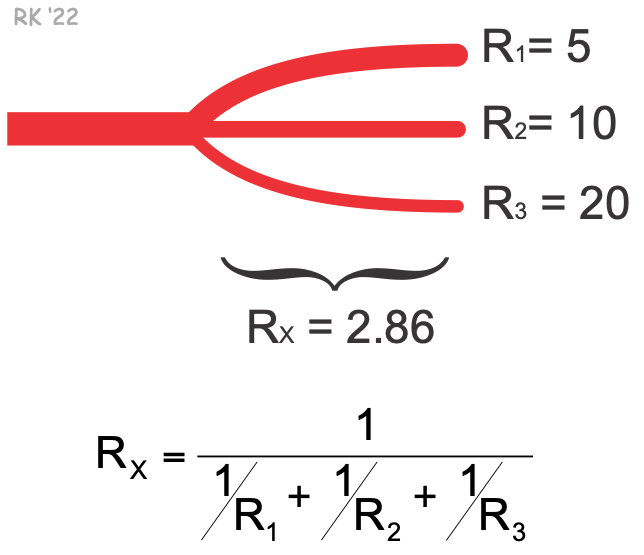# Parallel Resistance Calculations

Assume that a small artery is giving rise to three smaller arterioles, each parallel to the other, as shown below. If the resistance value for R1 = 5, R2 = 10 and R3 = 20, then the total resistance (Rx) for the three parallel arterioles comprising the segment would be 2.86 using the equation for calculating parallel resistance.This calculation shows two important principles regarding the parallel arrangement of blood vessels:

1. The total resistance of a network of parallel vessels is less than the resistance of the vessel having the lowest resistance. Therefore, a parallel arrangement of vessels reduces resistance to blood flow. That is why capillaries, which have the highest resistance of individual vessels because of their small diameter, comprise only a small portion of the total vascular resistance of an organ or microvascular network.
2. When there are many parallel vessels, changing the resistance of a few of these vessels will have a relatively small effect on total resistance for the segment.

Revised 12/15/2022

DISCLAIMER: These materials are for educational purposes only, and are not a source of medical decision-making advice.